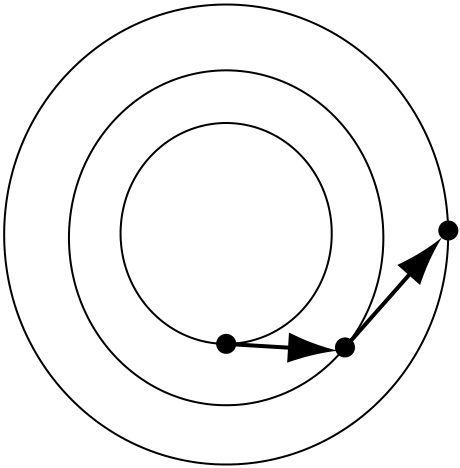Rating : ⭐⭐⭐⭐⭐
Price : \$10.99
Language:EN
Pages: 2

# Verlet method velocity verlet with the previous verlet methods

618 Chapter 13 Rigid Body Dynamics

position calculation:

X(t + h) = X(t) + hv(t) +h2 2a(t)

v(t + h) = v(t) + h/2[a(t) + a(t + h)]13.3 Numerical Integration 619

Implicit methods make use of quantities from the next time step:

yi+1 = yi + hi yi+1

x2 x1

Figure 13.7 Implicit Euler. The arrows point backwards to indicate that we are getting the derivative from the next time step.

How It Works